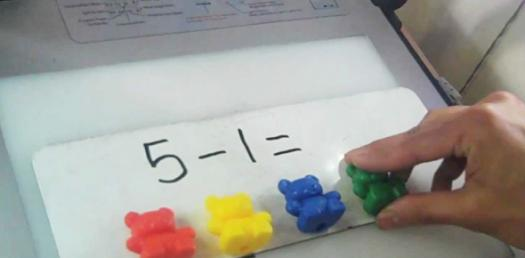# Addition Trivia Quiz: Math Skills Test For Beginners!

48 Questions | Attempts: 1967SettingsIn our quiz we look to practice the concepts of math learnt by beginners. The quiz looks to give you skills necessary to solve these problems. These questions will give you the skills required as a beginner before dive into solving more complex math problems. Do give it a try and see how easy it is to solve these problems.

• 1.
2+3=
• 2.
5+1=
• 3.
4+2=
• 4.
1+2=
• 5.
0+6=
• 6.
4+0=
• 7.
4+1=
• 8.
3+3=
• 9.
1+0=
• 10.
2+3=
• 11.
4+1=
• 12.
1+3=
• 13.
4+2=
• 14.
2+2=
• 15.
1+4=
• 16.
2+3=
• 17.
0+2=
• 18.
4+0=
• 19.
5+1=
• 20.
3+1=
• 21.
0+5=
• 22.
2+3=
• 23.
3+2=
• 24.
4+1=
• 25.
6+0=

## Related TopicsBack to top
×

Wait!
Here's an interesting quiz for you.